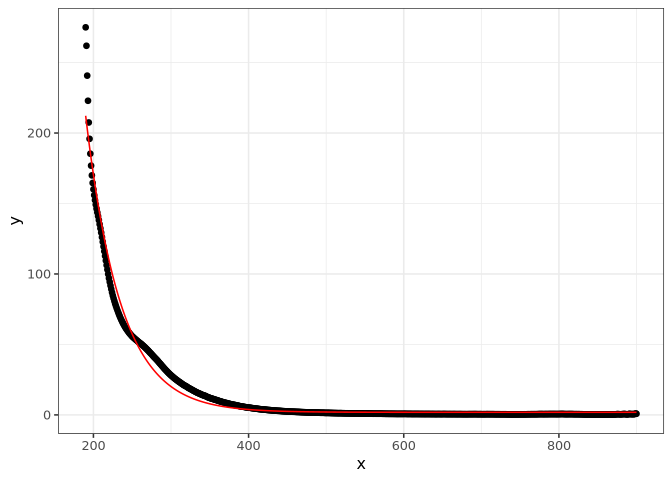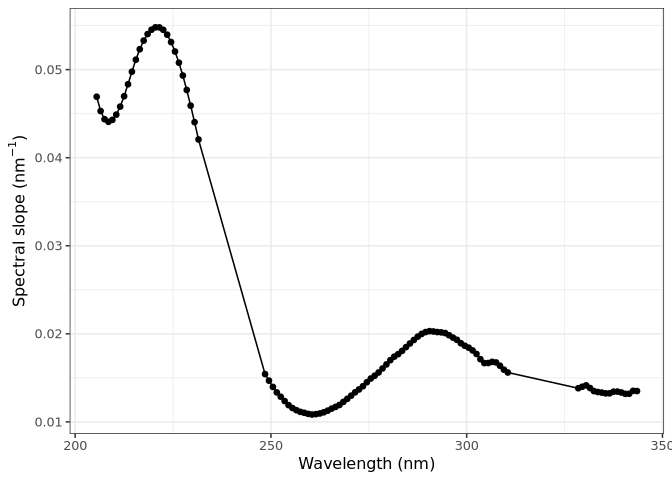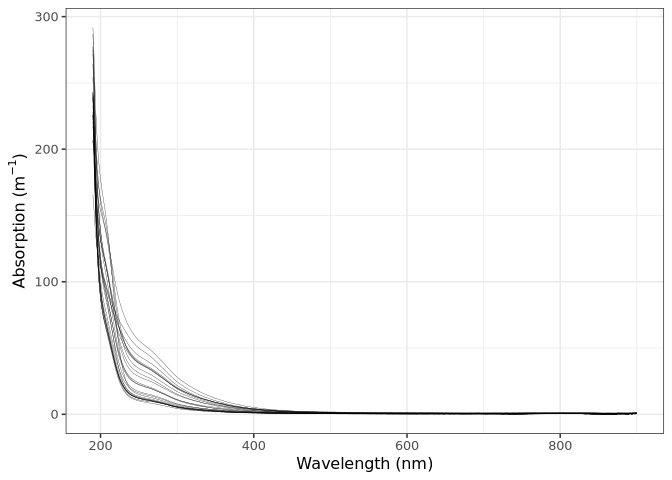## cdomThe cdom package implements various functions used to model and calculate metrics from absorption spectra of chromophotic dissolved organic matter (CDOM).

This package provides:

1. Simple wrappers to calculate common metrics found in the literature.
• The spectral curve (Loiselle et al. 2009).
• The slope ratio (Sr) (Helms et al. 2008).
• The spectral slope (S) (Jerlov 1968; Lundgren 1976; Bricaud, Morel, and Prieur 1981).
2. The function to use the Gaussian decomposition approach proposed in Massicotte and Markager, (2015).

The package can be installed using the following command.

devtools::install_github("PMassicotte/cdom")

Please note that this is a developing version of the package for testing only. Please fill an issue when you find bugs.

All functions from the package start with the cdom_ prefix.

library(cdom)
ls("package:cdom")
##  "cdom_fit_exponential" "cdom_slope_ratio"     "cdom_spectral_curve"
##  "spectra"

# Examples

## The spectral slope (S)

The cdom_fit_exponential() function fits an exponential curve to CDOM data using the simple model proposed by Jerlov (1968), Lundgren (1976), Bricaud, Morel, and Prieur (1981).

a(\lambda) = a(\lambda0)e^{-S(\lambda - \lambda0)} + K
library(ggplot2)
library(cdom)
data("spectra")

fit <- cdom_fit_exponential(wl = spectra$wavelength, absorbance = spectra$spc3,
wl0 = 350,
startwl = 190,
endwl = 900)

ggplot(spectra, aes(x = wavelength, y = spc3)) +
geom_point() +
geom_line(aes(y = fit$data$.fitted), col = "red") +
xlab("Wavelength (nm)") +
ylab(expression(paste("Absorption (", m ^ {-1}, ")")))## The slope ratio (SR)

The cdom_slope_ratio() function calculates the slope ratio (SR) which is defined as: S275-295/S350-400. See Helms et al. (2008) for detailed information.

library(cdom)
data("spectra")

cdom_slope_ratio(spectra$wavelength, spectra$spc1)
##  1.325082

## The spectral curve

The cdom_spectral_curve() function generates the spectral curve using the slope of the linear regression between the natural log absorption spectrum and wavelengths over a sliding window of 21 nm interval (default) at 1 nm resolution. See Loiselle et al. (2009) for detailed information.

library(cdom)
data("spectra")

res <-  cdom_spectral_curve(wl = spectra$wavelength, absorbance = spectra$spc10,
interval = 21,
r2threshold = 0.98) # Maybe to restrictive...

ggplot(res, aes(x = wl, y = s)) +
geom_point() +
geom_line() +
xlab("Wavelength (nm)") +
ylab(expression(paste("Spectral slope (", nm ^ {-1}, ")")))# Data

A total 25 absorption spectra are provided in the package.

library(ggplot2)
library(tidyr)
data("spectra")

spectra <- gather(spectra, sample, absorption, -wavelength)

ggplot(spectra, aes(x = wavelength, y = absorption, group = sample)) +
geom_line(size = 0.1) +
xlab("Wavelength (nm)") +
ylab(expression(paste("Absorption (", m ^ {-1}, ")")))# How to cite the package

citation("cdom")
##
## To cite cdom in publications use:
##
##   Massicotte, P., and Markager, S. (2016). Using a Gaussian
##   decomposition approach to model absorption spectra of
##   chromophoric dissolved organic matter. Mar. Chem. 180, 24-32.
##   doi:10.1016/j.marchem.2016.01.008.
##
## A BibTeX entry for LaTeX users is
##
##   @Article{,
##     title = {Using a Gaussian decomposition approach to model absorption spectra of chromophoric dissolved organic matter},
##     author = {Philippe Massicotte and Stiig Markager},
##     journal = {Marine Chemistry},
##     year = {2016},
##     volume = {180},
##     pages = {24--32},
##   }